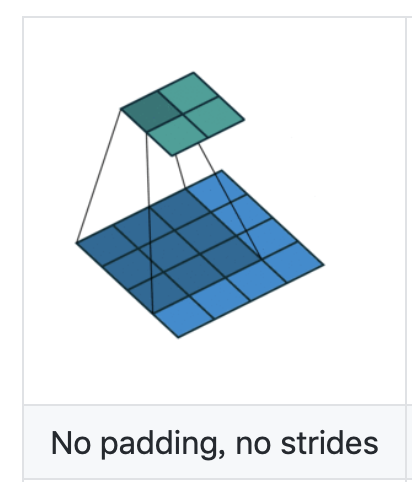# Why the default dilation value in Conv2d is 1?

class torch.nn.Conv2d(in_channels, out_channels, kernel_size, stride=1, padding=0, dilation=1, groups=1, bias=True)

The default value of dilation = 1, does it means all the conv2d without setting dilation = 0 will use dilation conv?

3 Likes

Dilation = 1 already means “no dilation”: 1-spacing = no gaps. I agree this convention is weird…

6 Likes

If you phrase it as “every `dilation`th element is used”, it may be easier to remember. When defining dilation (or any op in general) to me it seems natural to talk about what is used/done rather than what is skipped/not done.
So once you try to write down a formula, it probably is with this convention. Using it in code saves you from index juggling when putting formulas into code.

Of course, everyone has a different intuition about these things, but personally, I think the one pytorch implicitly suggests here can be useful.

Best regards

Thomas

3 Likes

dilation is similar to stride. stride=1 is not weird, and hence dilation=1 is not weird either10 Likes

Ok, please forgive my words, “weird” wasn’t the appropriate formulation. But it can be misunderstood by thinking that ‘=1’ implies there is a dilating dilation, hence the existence of this topic…

5 Likes

So in PyTorch, which image can represent the correct understanding?
Which image is the correct understanding of dilation value = 1?# Bottom one

1 Like

stride: step length for moving kernel.
dilation: step length for moving kernel element.

2 Likes